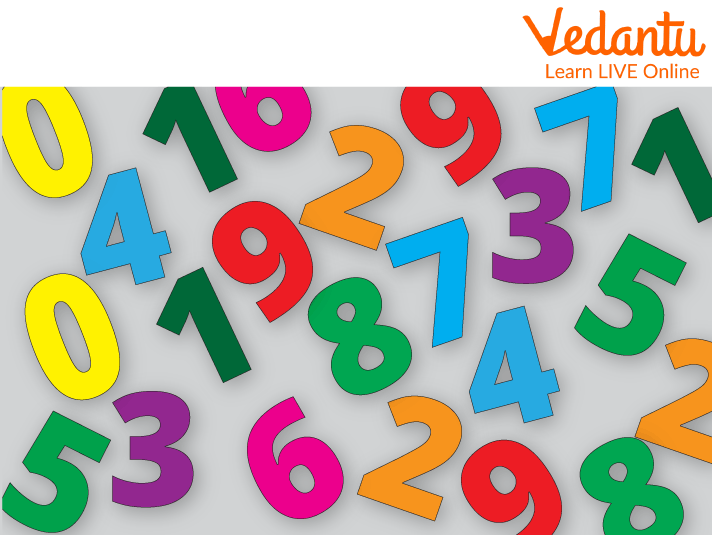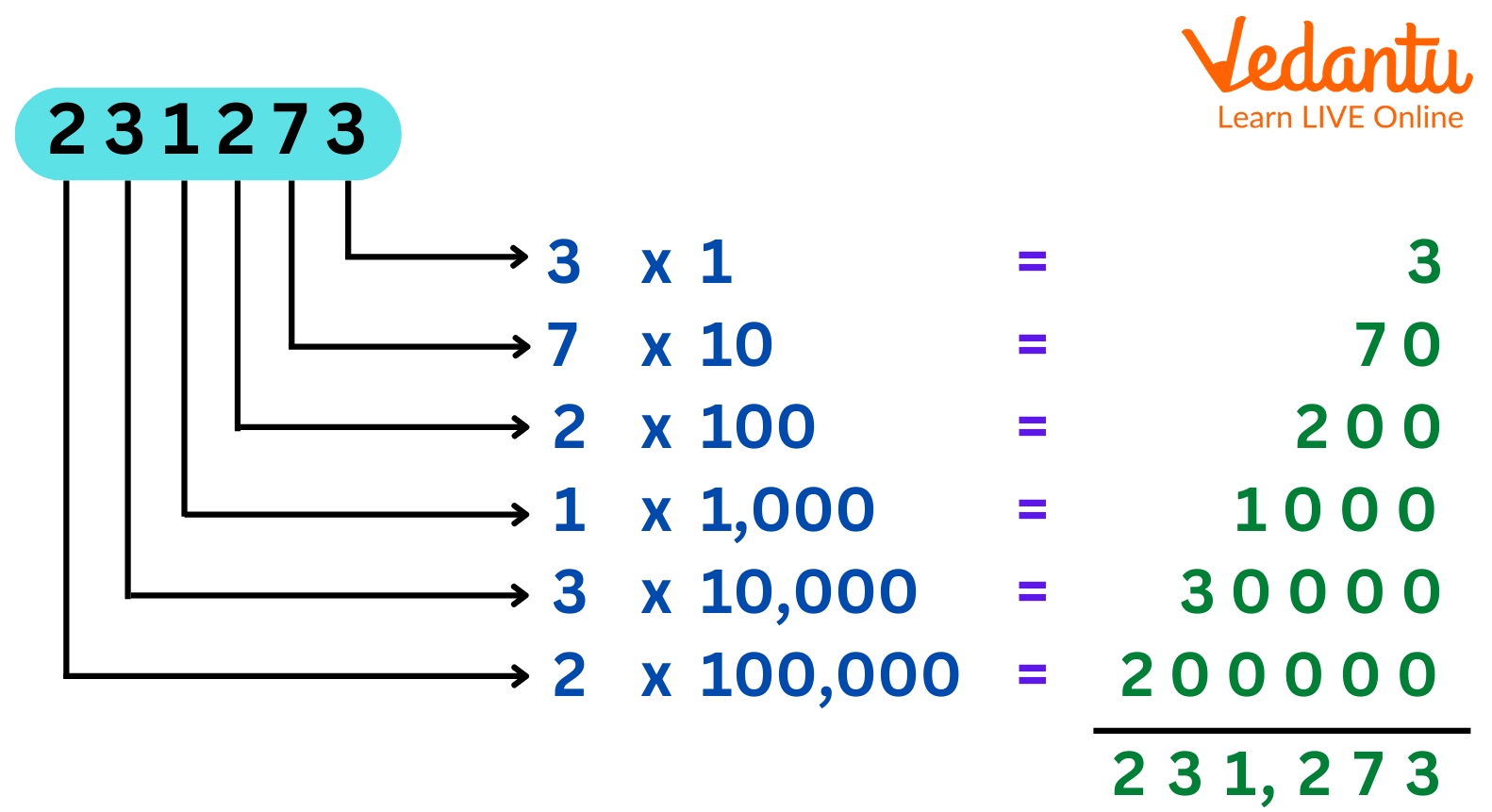Courses
Courses for Kids
Free study material
Free LIVE classes
More

# 11400 in WordsLIVE
Join Vedantu’s FREE Mastercalss

## Introduction of Number Words

The alphabetical representation of numbers is in number words. The English alphabet is used to write words that contain numbers. Numbers can be expressed using both words and numbers. These numbers are expressed as words, as the name suggests. For instance, we can create words of more value by using the numbers 1 through 10.

## What are Numbers?

A number is a numerical unit of measurement and labeling in mathematics. The natural numbers 1, 2, 3, 4, and so on are examples. Number words are the best way to express numbers. More commonly, specific numbers can be represented using symbols known as numerals; for instance, the numeral "5" stands in for the number five.Some Basic Numbers

## History of Numbers:

From the use of fingers and tally marks, maybe more than 40,000 years ago. Around 5000 or 6000 years ago in Mesopotamia, the first unambiguous notations for numerals are known to have appeared. Until the late 14th century, when the superior Hindu-Arabic numeral system proliferated and is still the most generally used method for representing numbers today, Roman numerals, which used a variety of Roman alphabet letters, were common in Europe.Famous Mathematician Aryabhatta

The success of the system depended on the sign for zero, which was invented by ancient Indian mathematicians in 500 AD.

## Expanded Form of Numbers:

The extended form of a number is obtained by categorizing numbers into groups based on place value, such as ones, tens, hundreds, thousands, ten thousand, and so forth. The number that is represented by adding the place values of each digit together is the enlarged version of the number.Expanded Form

## Place Value Chart for 11400:

 ONES 0 TENS 0 HUNDREDS 4 THOUSANDS 1 TEN THOUSANDS 1 LAKHS 0 TEN LAKHS 0

## How to Write 11400 in English Words:

11400 in words would be represented as ELEVEN THOUSAND AND FOUR HUNDRED.

Place value chart for 11400: To write the number 11400 in English, use the place value chart. We may easily write the numerical name 11400 by using the ones, tens, hundreds, thousands, and millions place values of each digit in the number. We note for 11400 that:

• The digit in place of one is 0

• The tenth digit is equal to zero.

• The hundredth-place digit is 4, thus.

• The thousandth digit is equal to one.

• The ten thousandth digit is equal to one.

• The digit at the hundred thousandth position is 0.

• The millionth digit is equal to zero.

• The ten millionth digit is equal to zero.

• The digit at the hundred millionth position is 0.

As a result, 11400 is represented as Eleven Thousand Four Hundred in words.

## Conclusion:

A number is a numerical unit of measurement and labeling in mathematics. Therefore 11400 in words is written as Eleven Thousand Four Hundred. Basic numerals are frequently grouped in a numeral system, which is an ordered manner to represent any number, because only a small number of symbols can be learned.

## Solved Examples:

1. What will be the expanded form of 11,400?

The expanded form of 11,400 will be

$11400=1 \times 10000+1 \times 1000+ 4 \times 1000 \times 1+0 \times 0$

2. Write 76,912 in words?

76,912 in words will be :

Seventy six thousand nine hundred and twelve.

3. Place value of 4 in 11400?

Place value of 4 in 11400 is 400.

Last updated date: 25th Sep 2023
Total views: 69.6k
Views today: 0.69k

## FAQs on 11400 in Words

1. What are the predecessor and successor ?

The two terms in mathematics that are most frequently used to describe series and sequences of whole numbers are predecessor and successor. The preceding numbers (those that precede) are referred to as the predecessor, and the following numbers are referred to as the successor (that appear just after).

2. What is the number system?

A number system is a logical way of writing numbers that uses digits or symbols to represent them.

3. Is 11400 a prime number?

No , 11400 is not a prime number as it is divisible by 2 , 3 , 4 , 5 , 10  and many more other numbers.

4. What is the hundredth digit?

The tenths place is represented by the first digit following the decimal. The hundredths place is represented by the digit that follows the decimal.